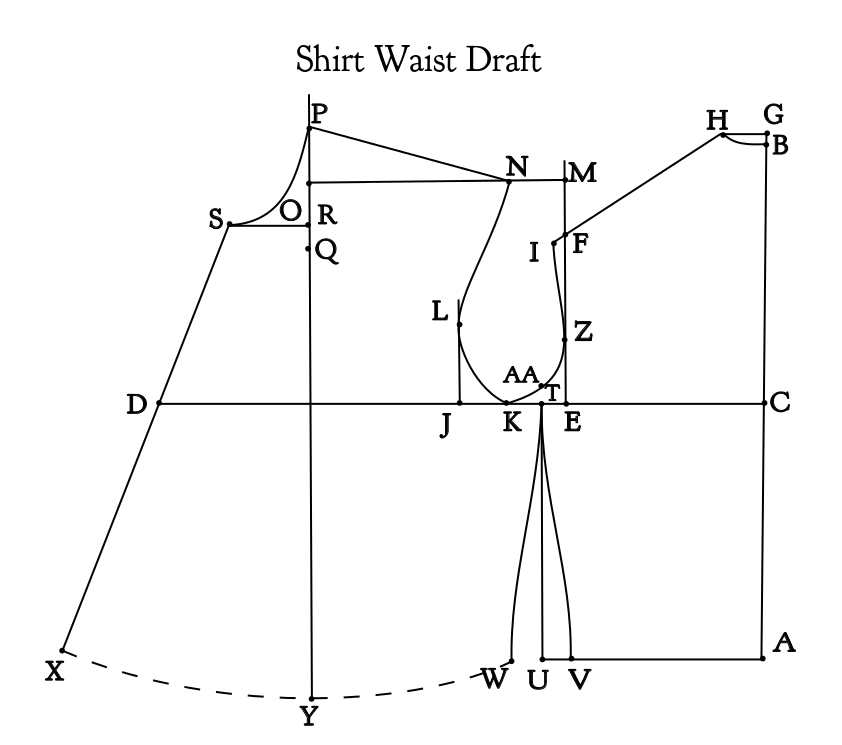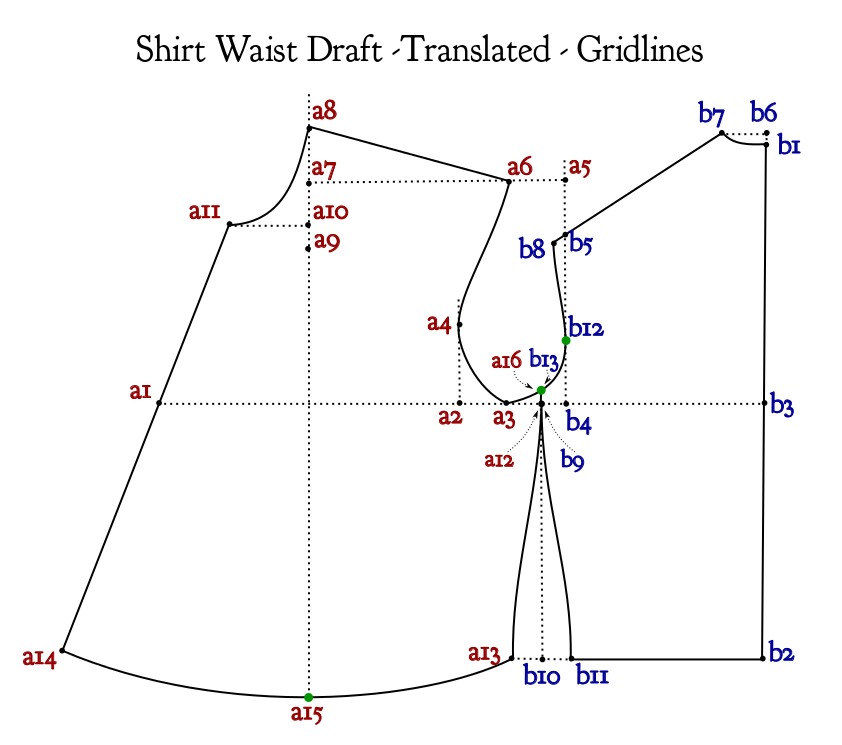# Creating a Pattern as an Inkscape Extension: Part 2

Continuation of Part 1

Here is the pattern with the new points added:STEP 4: RENAME THE POINTS
The next step is to convert each point into a naming convention. The pattern pieces are single capital letters. The Front Bodice will be pattern piece A, the Back Bodice pattern piece B.  All points for the Front Bodice should begin with  a lowercase ‘a’, and for the Back Bodice a lowercase ‘b’, followed by digits. Map out the conversion following the order the points are calculated:

• B –> b1
• A –> b2
• C –> b3
•                             D –> a1
• E –> b4
• F –> b5
• G –> b6
• H –> b7
• I –> b8
•                              J –> a2
•                              K –> a3
•                              L –> a4
•                              M –> a5       # M – doesn’t matter which pattern piece
•                              N –> a6
•                              O –> a7
•                              P –> a8
•                              Q –> a9
•                              R –> a10
•                              S –> a11
• T –> b9               T –> a12      # T is shared between front & back
• U–> b10                                      # U – doesn’t matter which pattern piece
• V –> b11
•                              W –> a13
•                              X –> a14
•                              Y –> a15
• Z –> b12
• AA –> b13          AA –> a16     # AA is shared between front & back

Now replace the old letters with the new variable names.   Note that point T is replaced with two points, a12 and b9, and point AA is also replaced with two points, a16 and b13.

The solid reference lines makes it difficult to clearly see the two front and back bodices. The front waistline may have been drawn as a dashed line to indicate that it changes for each person.  Here is the drawing with the reference lines changed to dashes, and the waistline changed to solid, and all the points renamed to match the naming convention:STEP 5: SUBSTITUTE THE NEW VARIABLE NAMES INTO THE FORMULAS

b1=0, 0
b2=downPoint(b1, front_waist_length)
b3=upPoint(b2, side)
a1=leftPoint(b3, bust_circumference/2.0)
b4=leftPoint(b3, across_back/2.0)
b5=upPoint(b4, armscye_circumference/3.0)
b6=upPoint(b1, 0.5*IN)
b7=leftPoint(b6,1.5*IN)
b8=intersectLineAtLength(b5,b7, -0.5*IN)
a2=leftPoint(b4, armscye_circumference/4.0)
a3=midPoint(a2,b4)
a4=upPoint(a2.x, 2.5*IN)
a5=upPoint(b5.x, 1.5*IN)
a6=leftPoint(a5, 2*IN)
a7=leftPoint(a5, distance(b7,b8))
a8=a7.x, b3.y-upper_front_height
a9=downPoint(a8, neck_circumference/4.0)
a10=upPoint(a9, 0.5*IN)
a11=leftPoint(a10, (neck_circumference/6.0)+0.25*IN )
b9=leftPoint(b4, 1*IN)
a12=b9
b10=downPoint(b9, side)
b11=rightPoint(b10, 1*IN)
a13=leftPoint(b10, 1*IN)
a14=intersectLineAtLength(a11, a1, front_waist_length)
a15=downPoint(a8, distance(a8, a14))
b12 = downPoint(b5, (2/3.0)*distance(b5, b4))
curve_a3b12=pointList(a3, b12.c1, b12.c2, b12)
b13=intersectLineCurve(b9,b10,curve_a3b12)
a15=b13

Creating a Pattern with an Inkscape Extension will continue in the next blog post